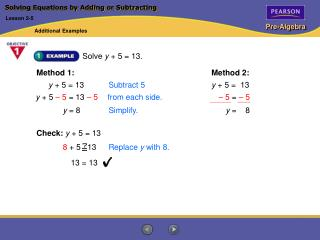DownloadDownload PresentationSolving Equations by Adding or Subtracting

# Solving Equations by Adding or Subtracting

Download Presentation## Solving Equations by Adding or Subtracting

- - - - - - - - - - - - - - - - - - - - - - - - - - - E N D - - - - - - - - - - - - - - - - - - - - - - - - - - -
##### Presentation Transcript

1. y + 5 = 13 Subtract 5 y + 5 – 5 = 13 – 5from each side. – 5 = – 5 Check:y + 5 = 13 8 + 5 13 Replace y with 8. 13 = 13 Solving Equations by Adding or Subtracting Lesson 2-5 Additional Examples Solve y + 5 = 13. Method 1: Method 2: y + 5 = 13 y = 8 Simplify. y = 8

2. Words target number is 250 plus number to buy Let x = number to buy. 327 = 250 + x Equation Solving Equations by Adding or Subtracting Lesson 2-5 Additional Examples Larissa wants to increase the number of books in her collection to 327 books. She has 250 books now. Find the number of books she needs to buy.

3. Check:  Is the answer reasonable? 250 plus the number of books bought should be a total collection of 327. 250 + 77 = 327 Solving Equations by Adding or Subtracting Lesson 2-5 Additional Examples (continued) 327 = 250 + x 327 = x + 250 Use the Commutative Property of Addition. 327 – 250 = x + 250 – 250Subtract 250 from each side. 77 = xSimplify. Larissa needs to buy 77 more books.

4. Solving Equations by Adding or Subtracting Lesson 2-5 Additional Examples Solve c – 23 = – 40. c – 23 = – 40 c – 23 + 23 = – 40 + 23Add 23 to each side. c = –17 Simplify.

5. Words cost of CD player was \$115 less than cost of DVD player t Let = cost of the DVD player. Equation 78 = t – 115 Solving Equations by Adding or Subtracting Lesson 2-5 Additional Examples Marcy’s CD player cost \$115 less than her DVD player. Her CD player cost \$78. How much did her DVD player cost? 78 = t – 115 Write an equation. 78 + 115 = t – 115 + 115 Add 115 to each side. 193 = tSimplify. Marcy’s DVD player cost \$193.